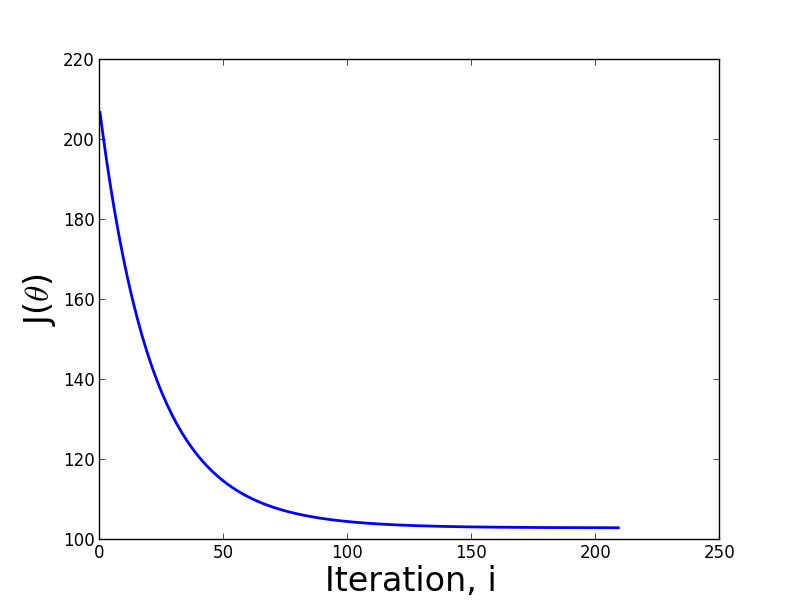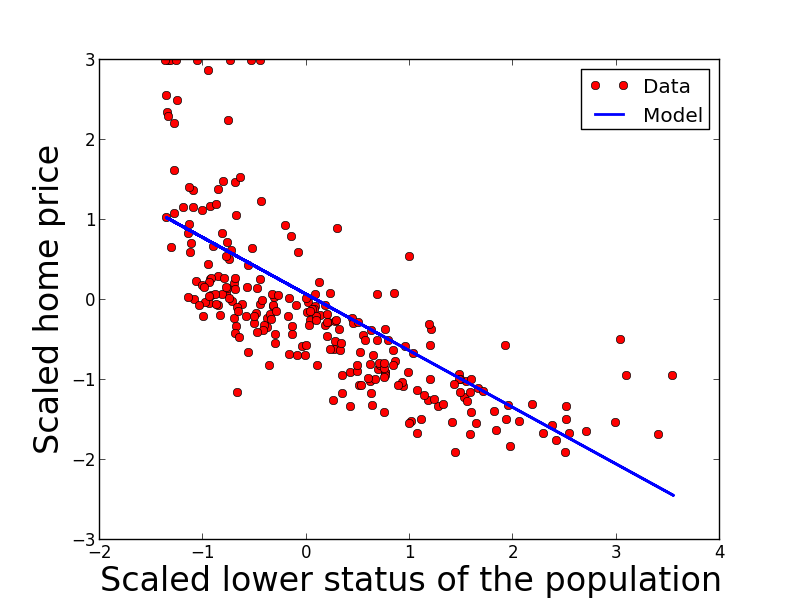# Machine Learning With Gradient Descent

In this post I'll provide a cursory introduction to the gradient descent algorithm and use it to fit a linear regression model. I'll then analyze its performance and discuss implementation details with examples given in Python.

Before delving into gradient descent, we need to introduce a bit of what's more or less standard notation. A supervised machine learning problem usually involves trying to estimate a function composed of a set of parmeters $\theta$, that maps a vector of features, $x$, onto a scalar target, $y$. The set of feature/target pairs are indexed using a subscript such that the $i^{th}$ observation is referred to as $(x_i,y_i)$.

Gradient descent is a simple, principled optimization algorithm used to choose parameter values in a variety of discriminative and generative predictive models. The algorithm works by efficiently searching the parameter space according to the following rule:

\begin{aligned} \theta := \theta -\alpha \frac{\delta}{\delta \theta}J(\theta). \end{aligned}

In the realm of machine learning, $J(\theta)$ is known as the cost function and $\alpha$ is the learning rate, a free parameter. When the cost function is expressed as least squares \begin{aligned} J(\theta) = \frac{1}{2}\sum_{i=1}^m (y_i - h_\theta(x_i))^2, \end{aligned} where $m$ is the total number of training examples, $h_\theta(x_i)$ is the hypothesis function, $\frac{\delta}{\delta \theta}J(\theta)$ is equal to \begin{aligned} \sum_{i=1}^m(y_i-h_\theta(x_i))x_i. \end{aligned} The gradient descent algorithm can now be expressed as \begin{aligned} \theta := \theta -\alpha \sum_{i=1}^m(y_i-h_\theta(x_i))x_i. \end{aligned} After each iteration the algorithm updates the parameters $\theta$ by iterating over all $m$ examples. This form of gradient is known as batch mode. We'll discuss other forms of gradient descent at the end of this post.

#### Linear regression

Linear regression fits a linear model, \begin{aligned} y = \theta_0 + \theta_1 x_1 + \dots + \theta_n x_n \\ \end{aligned} to a set features and targets. Our objective is find the set of parameters $\theta$ that do the best job predicting $y$. As we've already mentioned, to estimate these parameters we'll use gradient descent. To begin we define the hypothesis function as \begin{aligned} h_\theta(x) = \sum_{i=1}^n \theta_i x_i = \theta^{T}x. \end{aligned} Using $h_\theta(x)$, the resulting gradient descent update rule is \begin{aligned} \theta := \theta -\alpha (y_i-\theta^{T}x_i)x_i. \end{aligned} To estimate $\theta$, the data is scanned until the algorithm converges according to some criteria, which we'll discuss in the implementation.

#### Implementation

To implement linear regression using gradient descent, a data set is required. Since our implementation is in Python, we'll use the housing data contained in the sci-kit learn library. The complete code base used for this post can be found here.

Prior to running gradient descent its always a good idea to scale and center features in order to allow gradient descent to give equal weightings to each. We'll also split the data into training and test sets and use the training set to to fit our model and the test set to measure how well it performs a making predictions. A model's performance on unseen data is known as generalization error.


#scale data, split into training and test sets
features = sklearn.preprocessing.scale(data['data'][:,12])
features = np.array([np.append(1,i) for i in features])
y = sklearn.preprocessing.scale(data['target'])
train = features[:features.shape/2]
ytrain = y[:y.shape/2]
test = features[features.shape/2:]
ytest = y[y.shape/2:]

#learning rate
a = 0.0001

#error difference, convergence criteria
ep=0.001



Next we initialize the algorithm's state by creating a random vector of intial values for theta, and computing its cost, $J(\theta)$.


def bgd(a, x, y, ep=0.001, max_iter=10000):
### Initialize the algorithm state ###
cvg = False
m = 0
n = x.shape

#intialize the parameter/weight vector
theta = np.random.random(x.shape)

#for each training example, compute the gradient
z = [(y[i]-np.dot(t,x[i]))*x[i] for i in range(n)]

#update the parameters
t = t + a * sum(z)

#compute the total error, J(theta)
pe = sum([(y[i]-np.dot(t,x[i]))**2 for i in range(n)])



The core portion of the gradient descent algorithm is shown below. The code loops until the error between passes over the data is smaller than a pre-defined value or the maximum number of iterations is exceeded. It then returns the vector of parameters $\theta$.


### Iterate until convergence or max iterations ###
while not cvg:
#compute the value of the d/d_theta j(theta)
z = [(y[i]-np.dot(t,x[i]))*x[i] for i in range(n)]

#update theta parameters
theta = theta + a*sum(z)

#calculate the mean squared error
e = sum([(y[i]-np.dot(t,x[i]))**2 for i in range(n)])

#if the difference in error is less than ep, converged
if abs(pe-e) <= ep:
print '*** Converged, iterations: ', m, ' ***'
cvg = True

#update error and iteration counter
pe = e
m+=1

#if iterations exceeded, break
if m == max_iter:
print '*** Max interactions exceeded ***'
cvg = True

return theta



To measure the convergence of the algorithm we can examine the rate at which the cost function, $J(\theta)$ decreases. The figure below shows the value of $J(\theta)$ after each pass over the data set. In the early stages of parameter estimation the cost function is relatively large, but it decreases exponentially and finally tapers off around the $150^{th}$ pass.Analyzing a figure such as the one above is a good way to choose learning rate. If the algorithm does not exhibit a curve that is decreasing as a function of iterations ,such as the one above, the learning rate can be adjusted accordingly.

To validate that our algorithm converged and chose good values for $\theta$ we can use another library and compare results. In this example we're only using a single feature, so we'll use scipy's linear regression method.

Scipy gives us estimated parameter values of $(\theta_0, \theta_1)$ = (0.064, -0.718) and our gradient descent algorithm produces $(\theta_0, \theta_1)$ (0.069, -0.707) - in fairly good agreement.

There are a multitude of ways with which to measure the performance of a model, such as ROC curves, precision-recall plots, and k-fold cross validation. Each method has their own set of trade-offs and one should choose an appropriate measure based on their own problem constraints. Since the focus of this post is on gradient descent and not on model performance measures, we'll use visual inspection to get a sense of how well it performs at making predictions. The figure below plots data and predictions using the test set of features and targets.#### Implementation details

Gradient descent is sensitive to the values of the data and as a result, multi- dimensional data should be centered and scaled.

In this example we fixed the learning rate to a constant value; however in practice, it is often configured to decrease as a function of the state of the algorithm. Often times, an adaptive learning rate can speed up convergence times by making larger changes to the values of $\theta$ in early iterations and smaller changes later.

There are many non-trivial subtleties associated with implementing the version of gradient descent we have presented here. Perhaps the most important is the size of the data set. When the input data is large, the algorithm suffers from having to examine all the data at each parameter update. When the data is too large to fit into memory and must reside on disk, batch gradient descent can quickly become useless because its performance is limited by disk I/O.

To work around convergence time and input data size, other variants of gradient descent have been developed. Two of the most popular are stochastic gradient and mini-batch descent. Stochastic gradient descent performs parameter updates after observing each example and is often useful in online settings. Mini-batch gradient descent performs parameter updates on subsets of the data, thus providing a balance between stochastic and batch approaches.

#### Implementations

There are a variety of gradient descent implementations. Two popular libraries used in the research community are

## Tags

machine learning data science

Archives